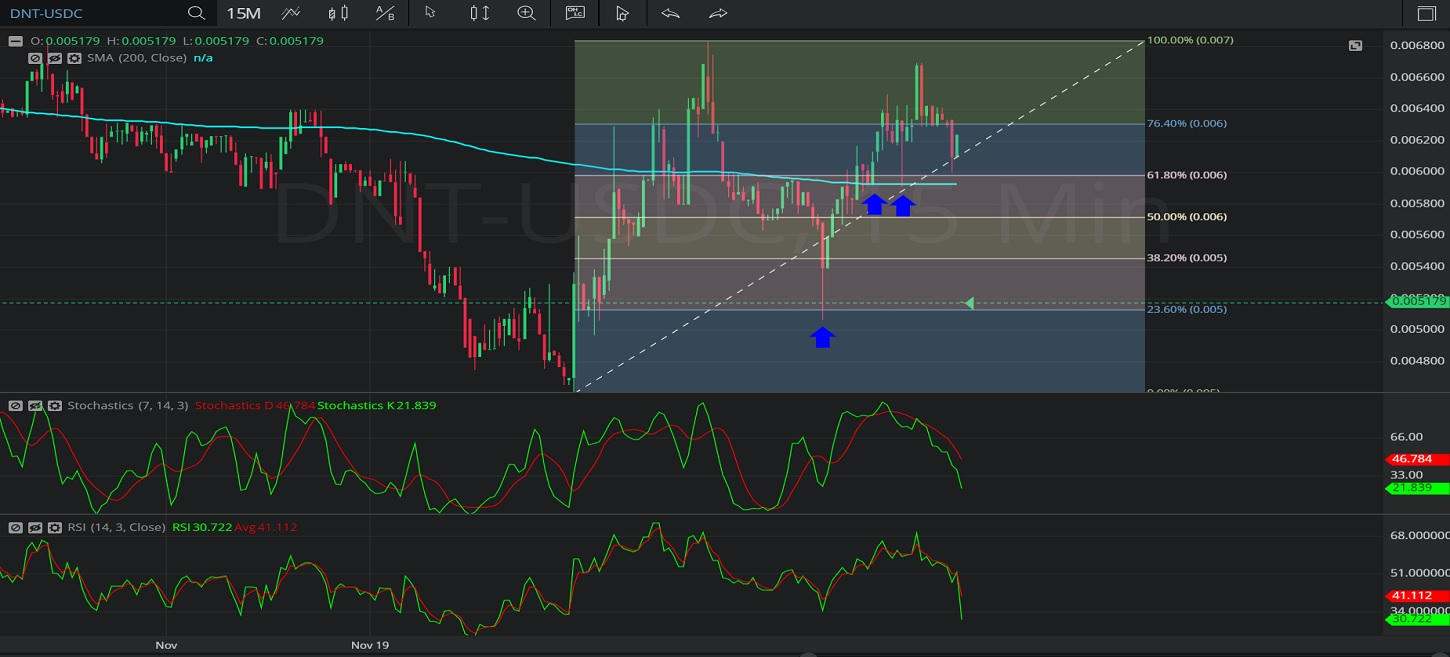Hot Topics

## 12 January 2020 - DNT/ USDC – 15-minuteIn this 15-minute chart of district0x/ US dollar Coin (DNT/ USDC), there is an appreciating range from 0.004592 to 0.006828.

We can observe that the market then depreciated to the 0.005072 level (identified by the first blue up arrow), just below the 0.00511969 level that represents the 76.4% retracement of the appreciating range.

We then see that the market appreciated to the 0.006697 area.  Prior to reaching that level, bids emerged (identified by the two blue up arrows) around the 200-bar Simple Moving Average.

We can see that the market then depreciated to the 0.006 level, just above the 0.00597384 area that represents the 38.2% retracement of the appreciating range.

Using RSI, we can observe that the slope of RSI (14) and the slope of RSI Average (3) turned positive after the market traded as low as the 0.005072 level. We can also observe that RSI (14) crossed above RSI Average (3). We can see that the slope of RSI (14) and the slope of RSI Average (3) are now negative and that RSI Average (3) is currently indicating above RSI (14), suggesting additional price depreciation.

Using Slow Stochastics, we can observe that the slope of Stochastics K bullishly turned positive after the market traded as low as the 0.005072 level.  We can also see that Stochastics K bullishly moved above Stochastics D before the slope of Stochastics D turned positive. We can see that the slope of Stochastics D bearishly turned negative after the market traded as high as the 0.006697 level, suggesting price depreciation is likely.

Stay on top of the cryptocurrency market with CryptoDaily’s trading charts!

https://charts.cryptodaily.net/